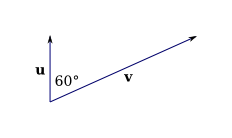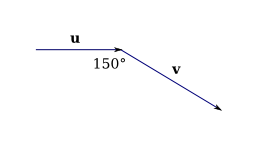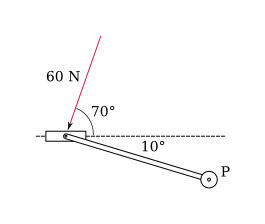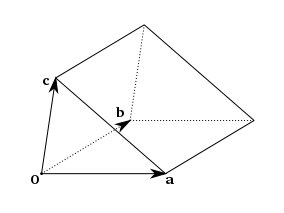Cross Product

(requires JavaScript)

1. Determine whether each expression is meaningful. If it is, state whether it's a vector or a scalar. If not, explain why.
1. $\mathbf{a}•\left(\mathbf{b}×\mathbf{c}\right)$
2. $\mathbf{a}×\left(\mathbf{b}•\mathbf{c}\right)$
3. $\mathbf{a}×\left(\mathbf{b}×\mathbf{c}\right)$
4. $\left(\mathbf{a}•\mathbf{b}\right)×\mathbf{c}$
5. $\left(\mathbf{a}•\mathbf{b}\right)×\left(\mathbf{c}•\mathbf{d}\right)$
6. $\left(\mathbf{a}×\mathbf{b}\right)•\left(\mathbf{c}×\mathbf{d}\right)$
Scalar, meaningless, vector, meaningless, meaningless, scalar.
2. If $\left|\mathbf{u}\right|=5$ and $\left|\mathbf{v}\right|=10$, find $\left|\mathbf{u}×\mathbf{v}\right|$ and determine whether $\mathbf{u}×\mathbf{v}$ is directed into the page or out of the page.$25\sqrt{3}$, into the page
3. If $\left|\mathbf{u}\right|=6$ and $\left|\mathbf{v}\right|=8$, find $\left|\mathbf{u}×\mathbf{v}\right|$ and determine whether $\mathbf{u}×\mathbf{v}$ is directed into the page or out of the page.$24$, into the page.
4. A bicycle is pushed by a foot with a $60$ N force as shown. The shaft of the pedal is $18$ cm long. Find the magnitude of the torque about $P$.$10.8\mathrm{sin}\left(80°\right)\approx 10.6$ Nm.
5. Let $\mathbf{a}=3\mathbf{i}+2\mathbf{j}+4\mathbf{k}$ and $\mathbf{b}=\mathbf{i}-2\mathbf{j}-3\mathbf{k}$. Find the cross product $\mathbf{a}×\mathbf{b}$ and verify that it is orthogonal to both $\mathbf{a}$ and $\mathbf{b}$.
$2\mathbf{i}+13\mathbf{j}-8\mathbf{k}$
6. Find two unit vectors orthogonal to both $\mathbf{i}+\mathbf{j}+\mathbf{k}$ and $2\mathbf{i}+\mathbf{k}$.
$±\frac{⟨1,1,-2⟩}{\sqrt{6}}$
7. Let $P=\left(2,1,5\right)$, $Q=\left(-1,3,4\right)$, and $R=\left(3,0,6\right)$.
1. Find the vector orthogonal to the plane containing the points $P$, $Q$, and $R$.
2. Find the area of triangle $PQR$.
1. $\stackrel{\to }{PQ}=⟨-3,2,-1⟩$ and $\stackrel{\to }{PR}=⟨1,-1,1⟩$, so $\mathbf{n}=\stackrel{\to }{PQ}×\stackrel{\to }{PR}=⟨1,2,1⟩$.
2. The area is $\frac{\left|\stackrel{\to }{PQ}×\stackrel{\to }{PR}\right|}{2}=\frac{\sqrt{6}}{2}$.
8. A wrench $30$ cm long lies along the positive $y\text{-axis}$ and grips the bolt at the origin. A force is applied in the direction $⟨0,3,-4⟩$ at the end of the wrench. Find the magnitude of the force needed to supply $100$ Nm of torque to the bolt.
Approx. $417$ N.
1. Let $P$ be a point not on the line $L$ that passes through the points $Q$ and $R$. Show that the distance $s$ from the point $P$ to the line $L$ is

$s=\frac{\left|\mathbf{a}×\mathbf{b}\right|}{\left|\mathbf{a}\right|}$,

2. where $\mathbf{a}=\stackrel{\to }{QR}$ and $\mathbf{b}=\stackrel{\to }{QP}$.
3. Use the formula in part (a) to find the distance from the point $P\left(1,1,1\right)$ to the line through the points $Q\left(0,6,8\right)$ and $R\left(-1,4,7\right)$.
9. Use cross product and dot product to find the volume of the shape shown in the figure if $\mathbf{a}=⟨7,1,1⟩$, $\mathbf{b}=⟨2,0,-4⟩$, and $\mathbf{c}=⟨1,5,-1⟩$. If the lines look parallel, they are.